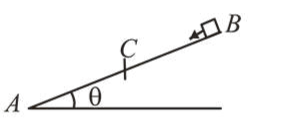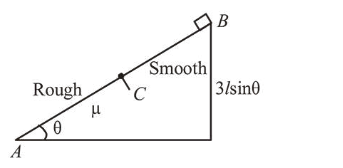# A small block starts slipping down from a point B on an inclined plane A B,`
Question:A small block starts slipping down from a point $B$ on an inclined plane $A B$, which is making an angle $\theta$ with the

horizontal section $B C$ is smooth and the remaining section $C A$ is rough with a coefficient of friction $\mu$. It is found that the block comes to rest as it reaches the bottom (point $A$ ) of the inclined plane. If $B C=2 A C$, the coefficient of friction is given by $\mu=k \tan \theta$. The value of $k$ is______

Solution:

(3)

If $A C=l$ then according to question, $B C=2 l$ and $A B=3 l$.Here, work done by all the forces is zero.

$W_{\text {friction }}+W_{m g}=0$

$m g(3 l) \sin \theta-\mu m g \cos \theta(l)=0$

$\Rightarrow \mu m g \cos \theta l=3 m g l \sin \theta$

$\Rightarrow \mu=3 \tan \theta=k \tan \theta$

$\therefore k=3$